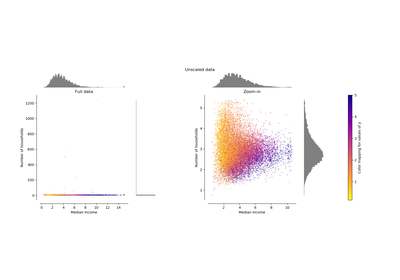# `sklearn.preprocessing`.Normalizer¶

class `sklearn.preprocessing.``Normalizer`(norm=’l2’, copy=True)[source]

Normalize samples individually to unit norm.

Each sample (i.e. each row of the data matrix) with at least one non zero component is rescaled independently of other samples so that its norm (l1 or l2) equals one.

This transformer is able to work both with dense numpy arrays and scipy.sparse matrix (use CSR format if you want to avoid the burden of a copy / conversion).

Scaling inputs to unit norms is a common operation for text classification or clustering for instance. For instance the dot product of two l2-normalized TF-IDF vectors is the cosine similarity of the vectors and is the base similarity metric for the Vector Space Model commonly used by the Information Retrieval community.

Read more in the User Guide.

Parameters: norm : ‘l1’, ‘l2’, or ‘max’, optional (‘l2’ by default) The norm to use to normalize each non zero sample. copy : boolean, optional, default True set to False to perform inplace row normalization and avoid a copy (if the input is already a numpy array or a scipy.sparse CSR matrix).

`normalize`
Equivalent function without the estimator API.

Notes

This estimator is stateless (besides constructor parameters), the fit method does nothing but is useful when used in a pipeline.

For a comparison of the different scalers, transformers, and normalizers, see examples/preprocessing/plot_all_scaling.py.

Methods

 `fit`(X[, y]) Do nothing and return the estimator unchanged `fit_transform`(X[, y]) Fit to data, then transform it. `get_params`([deep]) Get parameters for this estimator. `set_params`(**params) Set the parameters of this estimator. `transform`(X[, y, copy]) Scale each non zero row of X to unit norm
`__init__`(norm=’l2’, copy=True)[source]
`fit`(X, y=None)[source]

Do nothing and return the estimator unchanged

This method is just there to implement the usual API and hence work in pipelines.

Parameters: X : array-like
`fit_transform`(X, y=None, **fit_params)[source]

Fit to data, then transform it.

Fits transformer to X and y with optional parameters fit_params and returns a transformed version of X.

Parameters: X : numpy array of shape [n_samples, n_features] Training set. y : numpy array of shape [n_samples] Target values. X_new : numpy array of shape [n_samples, n_features_new] Transformed array.
`get_params`(deep=True)[source]

Get parameters for this estimator.

Parameters: deep : boolean, optional If True, will return the parameters for this estimator and contained subobjects that are estimators. params : mapping of string to any Parameter names mapped to their values.
`set_params`(**params)[source]

Set the parameters of this estimator.

The method works on simple estimators as well as on nested objects (such as pipelines). The latter have parameters of the form `<component>__<parameter>` so that it’s possible to update each component of a nested object.

Returns: self :
`transform`(X, y=’deprecated’, copy=None)[source]

Scale each non zero row of X to unit norm

Parameters: X : {array-like, sparse matrix}, shape [n_samples, n_features] The data to normalize, row by row. scipy.sparse matrices should be in CSR format to avoid an un-necessary copy. y : (ignored) Deprecated since version 0.19: This parameter will be removed in 0.21. copy : bool, optional (default: None) Copy the input X or not.

## Examples using `sklearn.preprocessing.Normalizer`¶Compare the effect of different scalers on data with outliersClustering text documents using k-means